ind2rgb_请教matlab中的rgb2ind函数问题 - CSDN

• ## rgb2ind函数

千次阅读 2017-05-26 14:27:14
rgb2ind函数对于减少RGB图像的颜色数是非常有用的。 源码：对彩色图像进行索引图像转化并抖动 f = imread('./face_2.jpg'); [x,map] = rgb2ind(f,8,'nodither');%非抖动处理 subplot(1,2,1),imshow(x,map) [x1,...
rgb2ind函数对于减少RGB图像的颜色数是非常有用的。
源码：对彩色图像进行索引图像转化并抖动
[x,map] = rgb2ind(f,8,'nodither');%非抖动处理
subplot(1,2,1),imshow(x,map)
[x1,map1] = rgb2ind(f,8,'dither');%抖动处理
subplot(1,2,2),imshow(x1,map1)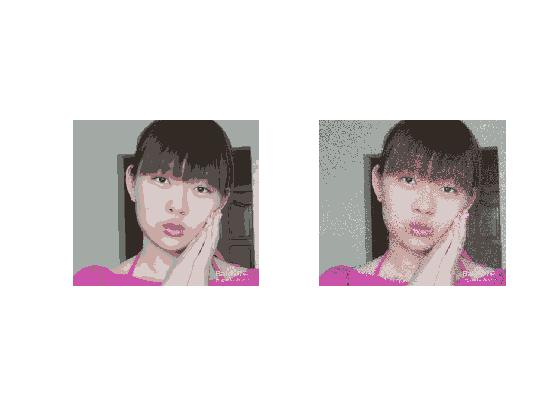可将图像确实是颜色的数量明显减少了，图一非常明显的显示了伪轮廓，图二对抖动处理后显示出了较好的色调，伪轮廓也明显减少了，
源码：抖动处理在灰度图像是非常明显的。
f = rgb2gray(f);
g1 = dither(f);%灰度图像抖动处理
subplot(1,2,1), imshow(f)
subplot(1,2,2), imshow(g1)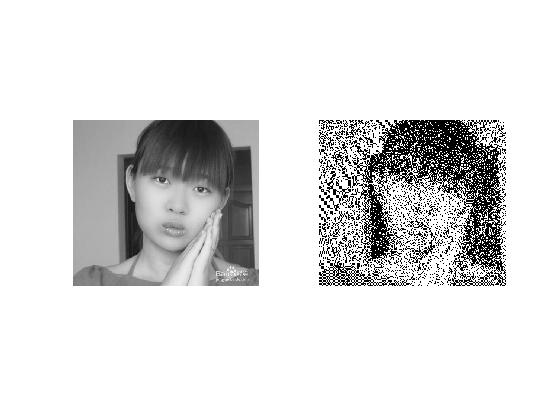展开全文索引图像 matlab
rgb图像化为灰度图是很容易的，matlab具有自带函数。
https://www.cnblogs.com/minisculestep/p/4878894.html
翻译如下：
问题：如何转变灰度图为rgb图像？
我使用下面脚本转换灰度图至rgb图像：
[im, map] = imread('frame1.jpg');
if(isempty(map))                        % image is RGB or grayscale
if(size(im, 3) == 1)                  % image is grayscale
im = cat(3, im, im, im);
end
else                                    % image is indexed
im = ind2rgb(im, map);
end

其中 frame1是灰度图。问题是:当我执行imshow(im)时，它仍然没有颜色，但是size(im)是144 176 3，我很困惑，我怎么才能得到有颜色的图像呢?
答：
所有的代码都在执行：
im=cat(3,im,im,im)

创建了144x176x3矩阵，其中im(:，:，1)与im(:，:，2)相同，im(:，:，3)相同。因此，红色、绿色和蓝色的分量是相同的，因此图像仍然是“灰度图”
您可以使用ind2rgb()应用各种颜色映射
map = hsv(256);                          % Or whatever colormap you want.
rgbImage = ind2rgb(im, map);             % im is a grayscale or indexed image.

使用颜色映射类型，因为您对感兴趣的颜色区域感兴趣，我认为任何颜色映射格式都足够了。例子:
   close all
clear
clc % just to clear things up
R=rgb2gray(I);                        % It is gray now
y=colormap(hot(110));
imwrite(R,y,'rgb.jpg','jpg');         % Re-change it to colored one
imshow('rgb.jpg');

如果您的初始图像是单色的，则无法确定原始颜色。你不能。如果你只有灰度图像就不是这样了。
可以使用cat()将灰度图像转换为RGB图像：
rgbImage = cat(3, grayImage, grayImage, grayImage);

当然，它会看起来所有的灰度，即使它是一个“真正的颜色”RGB图像-只是所有的“颜色”都是灰色的。您可以对灰度值应用伪颜色查找表，将每个灰度值映射到某个颜色，以得到一个多色图像，如下所示:
rgbImage = ind2rgb(grayImage, jet(256));

然而，这些颜色并不是虹膜的原始颜色，就像你用彩色相机拍下的那样。


展开全文matlab 图像处理
• 索引图像转换成真彩色图 [X,map] = imread('corn.tif'); //%索引图像X是uint8类型的415×312 数组....RGB = ind2rgb(X,map); //%将索引图像转换为 RGB 图像。结果为一个 double 类型的 415×312×3 数组。 disp(['Ra...
索引图像转换成真彩色图
[X,map] = imread('corn.tif');
//%索引图像X是uint8类型的415×312 数组.颜色图 cmap 是 double 类型的 256×3 矩阵，因此索引图像中有 256 种颜色。
RGB = ind2rgb(X,map);
//%将索引图像转换为 RGB 图像。结果为一个 double 类型的 415×312×3 数组。
disp(['Range of RGB image is [',num2str(min(RGB(:))),', ',num2str(max(RGB(:))),'].']);
//%检查 RGB 图像的值是否在 [0, 1] 范围内。
imshow(RGB);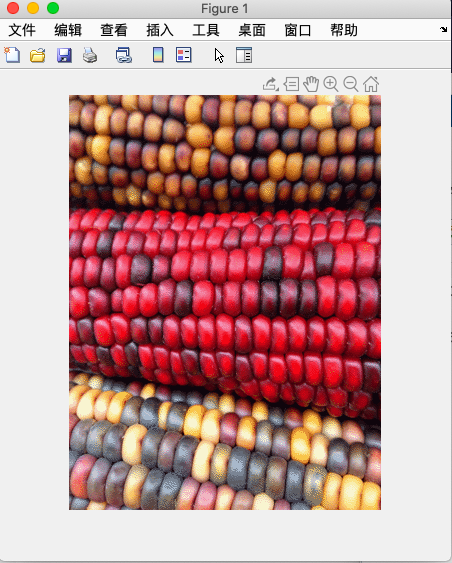ind2rgb()函数详解
语法
RGB = ind2rgb(X,map)

RGB = ind2rgb(X,map)将索引图像X和对应的颜色图map转换为RGB（真彩色）格式。
输入参数
X--索引图像 m×n整数矩阵

如果将 X 指定为整数数据类型的数组，则值 0 对应于颜色图 map 中的第一种颜色。对于包含 c 种颜色的颜色图，图像 X 的值会被裁剪到范围 [0, c-1] 内。
如果将 X 指定为 single 或 double 数据类型的数组，则值 1 对应于颜色图中的第一种颜色。对于包含 c 种颜色的颜色图，图像 X 的值会被裁剪到范围 [1, c] 内。

数据类型：single | double | uint8 | uint16
map--颜色图 c×3 的矩阵
与索引图像 X 相关联的颜色图，指定为由范围 [0, 1] 内的值组成的 c×3 矩阵。map 的每行都是一个三元素 RGB，指定颜色图的单种颜色的红、绿和蓝分量。
数据类型: double
输出参数
RGB--RGB图像 m×n×3 数值数组
RGB 图像，返回为由范围 [0, 1] 内的值组成的 m×n×3 数值数组。
数据类型：double


展开全文• 以下内容以书中内容为基础，加上自己的理解的表述，如有错误，望请批评指正MATLAB中的彩色图像的表示（图像格式）RGB图像所谓RGB图像，即为R（Red 红） G（Green绿） B（Blue蓝） 的灰度图（三个长宽一样的二维矩阵...
以下内容以书中内容为基础，加上自己的理解的表述，如有错误，望请批评指正

MATLAB中的彩色图像的表示（图像格式）

RGB图像

所谓RGB图像，即为R（Red 红） G（Green绿） B（Blue蓝） 的灰度图（三个长宽一样的二维矩阵）组成的图像比如以下这段代码

r = zeros(300, 300);
g = zeros(300, 300);
b = zeros(300, 300);
r(1:100, 1:100) = 1;
g(101:200, 101:200) = 1;
b(201:300, 201:300) = 1;
img = cat(3, r, g, b);
subplot(2, 2, 1), imshow(r), title('R');
subplot(2, 2, 2), imshow(g), title('G');
subplot(2, 2, 3), imshow(b), title('B');
subplot(2, 2, 4), imshow(img), title('RGB');

输入: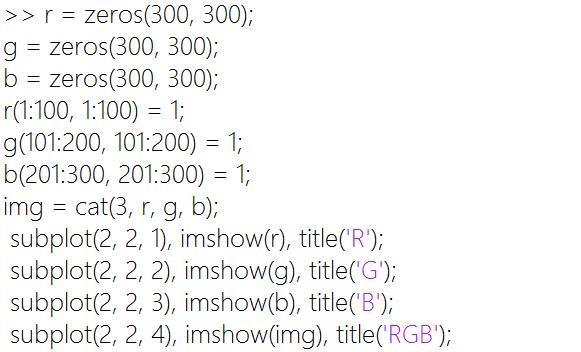输出: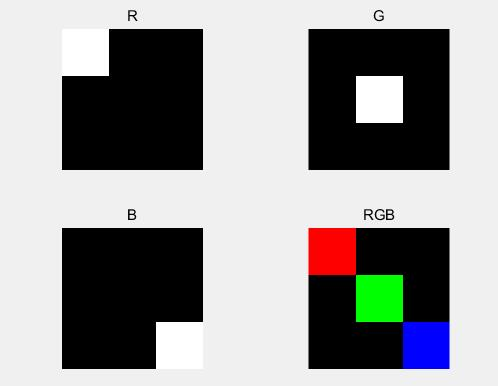可以看出 其实RGB 三张分别代表红绿蓝的灰度图 叠加在一起的图像。

索引图像

索引图像有两个分量: 一个整数数据矩阵X 和 一个彩色银蛇矩阵map。矩阵map是一个大小为m*3的double类数组，其值是区间[0， 1]上的浮点数。map的长度m等于其定义的颜色的个数。map存的是RGB三个分量。

f  = imread('onion.png');
[X, map] = rgb2ind(f, 2);
[X2, map2] = rgb2ind(f, 256);
[X3, map3] = rgb2ind(f, 65535);
subplot(2, 2, 1), imshow(f), title('原图');
subplot(2, 2, 2), imshow(X, map), title('索引图 2种颜色');
subplot(2, 2, 3), imshow(X2, map2), title('索引图 256种颜色');
subplot(2, 2, 4), imshow(X3, map3), title('索引图 65535种颜色');

输入: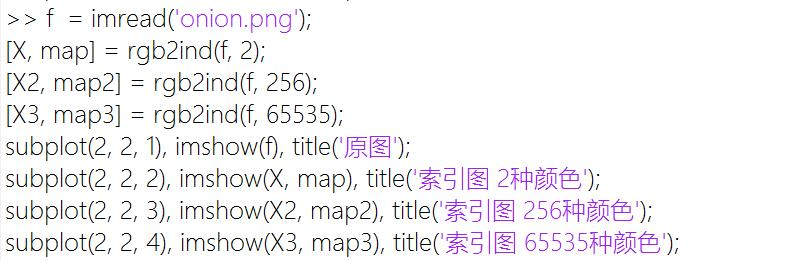输出: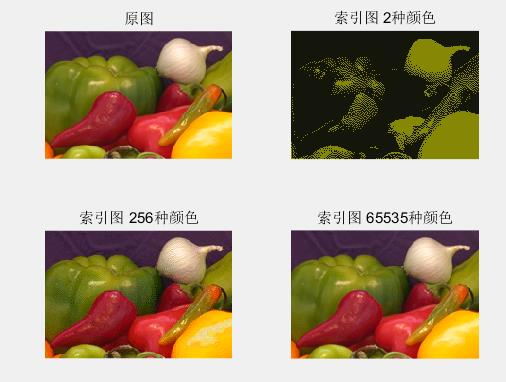处理RGB和索引图像的函数

* ind2rgb(X, map) 将索引图变为RGB图*

rgb2ind(image, n) 将RGB转为索引图，索引颜色数规定为n个，可以指定转换时运用什么方式进行颜色处理

有二种方式，一种为dither 抖动，另一种为nodither 不抖动。
抖动是什么意思呢，就是为了让图片看起来更加自然，每个像素的点会中和其邻域的点的颜色，这样就会显得这个点的像素颜色不那么突兀。

f = imread('onion.png');
subplot(2, 3, 1), imshow(f), title('原图');
subplot(2, 3, 4), bar([imhist(f(:, :, 1), 10), imhist(f(:, :, 2), 10), imhist(f(:, :, 3), 10)]), axis tight, title('原图的三色直方图');
[X, map] = rgb2ind(f, 256); % 默认为dither
subplot(2, 3, 2), imshow(ind2rgb(X, map)), title('dither');
f = ind2rgb(X, map);
subplot(2, 3, 5), bar([imhist(f(:, :, 1), 10), imhist(f(:, :, 2), 10), imhist(f(:, :, 3), 10)]), axis tight, title('dither的三色直方图');
[X, map] = rgb2ind(f, 256, 'nodither');
subplot(2, 3, 3), imshow(ind2rgb(X, map)), title('nodither');
f = ind2rgb(X, map);
subplot(2, 3, 6), bar([imhist(f(:, :, 1), 10), imhist(f(:, :, 2), 10), imhist(f(:, :, 3), 10)]), axis tight, title('nodither的三色直方图');

输入: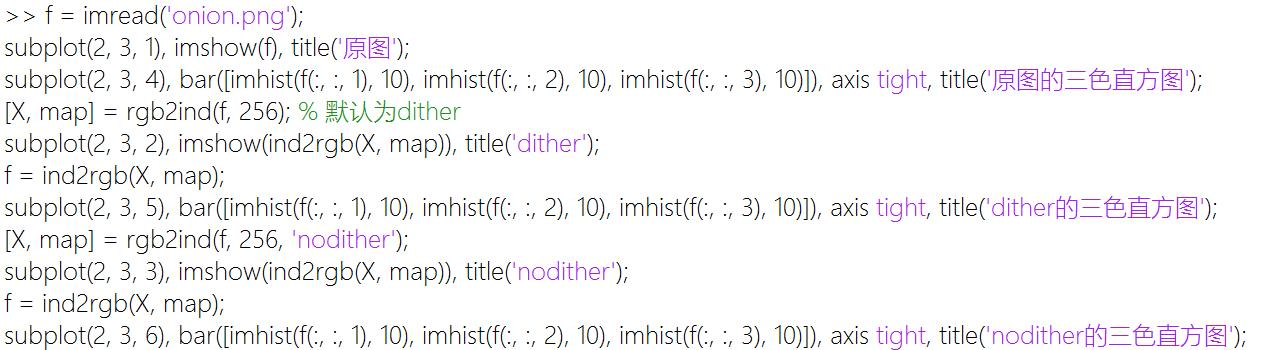输出: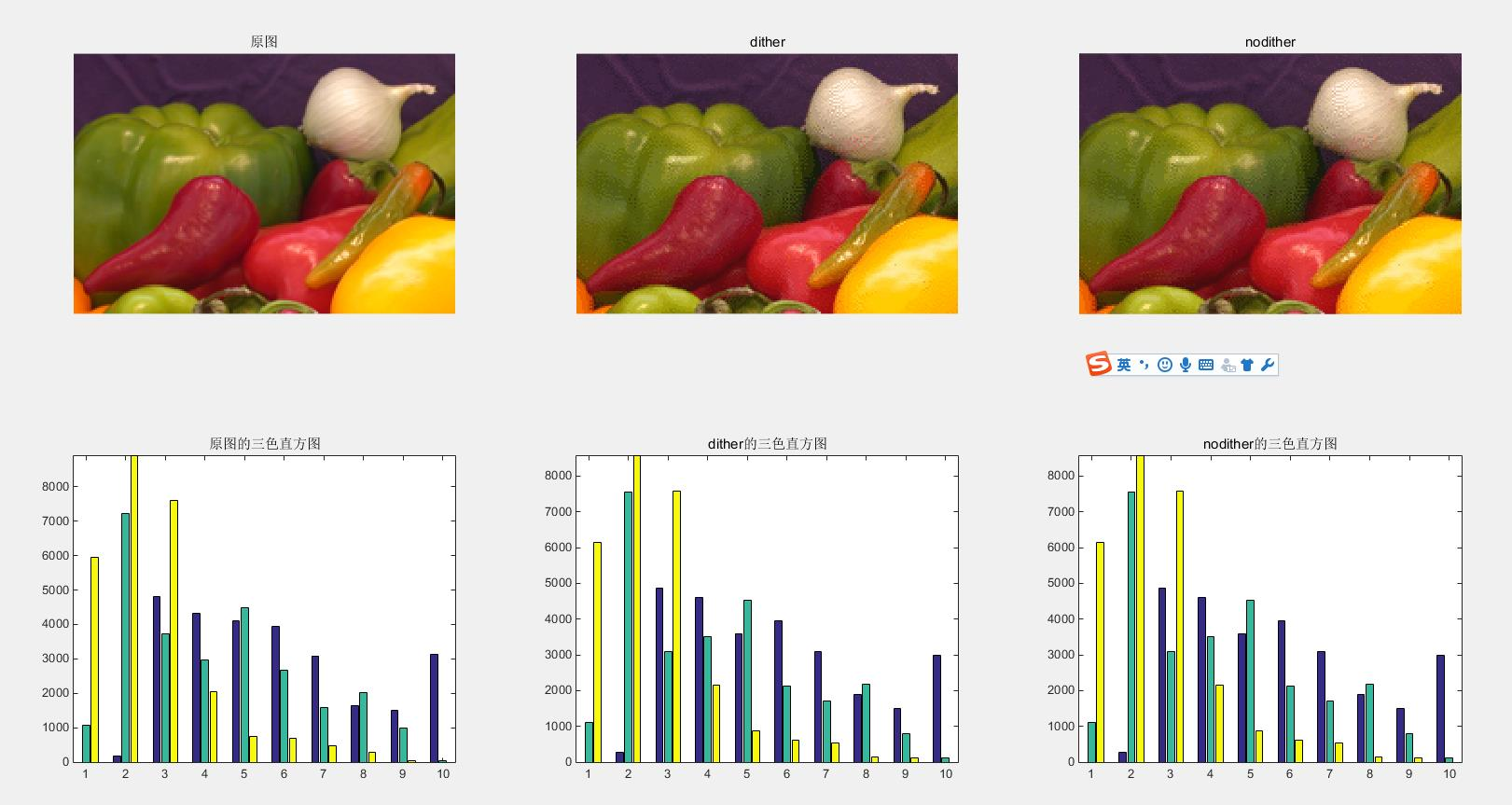dither(image) 该方法即为上面rgb2ind函数的dither方法，该函数处理灰度图效果最明显

f = imread('coins.png');
g = dither(f);
subplot(1, 2, 1), imshow(f), title('原图');
subplot(1, 2, 2), imshow(g), title('抖动出来的二值图');

输入:输出: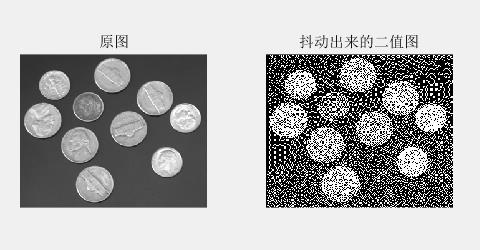展开全文matlab
• %使用rgb2ind函数减少一幅RGB图像中的色彩 f=imread('0602.tif') subplot(3,2,1),imshow(f),title('原图') [X1,map1]=rgb2ind(f,8,'nodither');%8为颜色的数目，nodither为不执行抖动处理 subplot(3,2,2),imshow(X1...
• 其中用到的工具箱函数： 函数 目的 dither 采用抖动从RGB图像创建索引图像 grayslice 采用阈值处理从灰度图像创建... rgb2ind 从RGB图像创建索引图像 ind2rgb 从索引图像创建RGB图像 rgb2gray 从RGB图像创rgb
• 如题，我用MATLAB配置了一张索引表，索引表为 8192*3，如何根据提供的矩阵（512*424）生成一张RGB图像（512*424*3）c++ matlab rgb
• 该函数将真彩色图像转换为索引图像， 由于RGB图像一个像素占用三个字节， 分别存储R、G、B分量的值，而索引图像一个像素占用一个字节。在将灰度图像转换为索引图像时， 是从3个字节映射到一个字节的关系。 通常有...计算机视觉
• Matab 灰度图 生成 伪彩色 图像 gray convert to pseudo color 原文出处： http://www.alecjacobson.com/weblog/?p=1655 ...In matlab you can view a grayscale image with: ...Which for my image
• 如truecolor转化为灰度图，可用函数rgb2gray即可实现，语法也比较简单。如下： grayImage=rgb2gray(srcImage); 加载完图片后可使用imfinfo()函数查看图像信息，通过colorType判断图像是什么类型，如为indexed即...ind2rgb 索引图像
• im = imread('color.jpg'); %彩色图 im = double(im); gray = imread('gray.jpg'); % 热力图 gray = double(gray); cmap = colormap(jet...rgb = ind2rgb(gray,cmap); rgb = rgb * 255; figure(1)
• ## 1.MATLAB图像处理基础知识

万次阅读 多人点赞 2017-05-06 14:20:57
在MATLAB中，基本数据结构为数列，大部分图像也是以数列的方式存储的，例如，包含1024列768行的彩色图像，在MATLAB中被存储为1024X768的矩阵；其中，矩阵的值为色彩值。这样就把图像变成了数列了，MATLAB就可以使用...
• ind的意思是index,即索引值，sub的意思是subscript，即下标，2就是to的意思。所以这个函数的意思是把矩阵中某个元素的索引值转换为下标。 索引值：矩阵中某个元素的索引值就是它在矩阵中排第几位的意思。 比如[1...matlab 函数 索引
• 数字图像处理的大作业， 通过GUI界面对图像进行一些简单的处理，有图像增强、滤波、裁剪、亮度调节、添加滤镜等功能 下面附上截图 需要的可以下载... 开始 打开 ...RGB2gray RGB2ind Ind2RGB Ind2gr...matlab大作业 数字图像 基本图像处理算法 简单易懂
• ## Matlab制作GIF动态图

万次阅读 2018-07-05 15:33:13
GIF动态图制作制作 clc clear close all filename='D:\\matlab_program\\Mycode\\fish1.gif'; % 输出路径+保存的文件名.gif for i=3374:3379 str = sprintf('D:\\matlab_program\\Mycode\\%d.png',i);...图片合成
• 彩色图像：每个像素由R、G、B三个分量表示，每个通道取值范围0~255。数据类型一般为8位无符号整形。 灰度图像：每个像素只有一个采样颜色的图像，这类图像通常显示为从最暗黑色到最亮的白色的灰度。...
• 简介 rgb2gray是matlab内部一种处理图像的函数，通过消除图像色调和饱和度信息同时保留亮度实现将将RGB图像或彩色图转换为灰度图像，即灰度化处理的功能，调用这个功能的格式是I = rgb2gray(RGB)，意思是将真彩色...
• ## 图像类型的转换

千次阅读 2015-04-29 13:31:54
1 RGB图像转换为灰度图像 在matlab中，将RGB图像装换为灰度图像，需要调用函数rgb2gray(),...在matlab中，可以直接调用rgb2ind()函数将真彩色图像转换为索引图像。最新的matlab版本(如matlabR2010a)中有均匀量化法、最
• ## MATLAB图像处理命令

万次阅读 2007-04-06 11:24:00
zzfrom: http://public.whut.edu.cn/lxy/mathmodel/Resource/course/Matlab%206.0图形图像处理函数详细参考.pdf1.applylut 功能: 在二进制... 语法: A = applylut(BW,lut) 举例 lut = makelut(sum(x(:)) == 4,2); BW1matlab 图像处理 filter fft
• 第六章 彩色图像处理 一、MATLAB中彩色图像的表示方法 1.1、RGB图像 ...fG = rgb_image(:, :, 2); fB = rgb_image(:, :, 3); 通常，能够从任何透视方向观察这个彩色立方体是很有用的，函数rgbcube图像处理 索引 rgb 数据
...